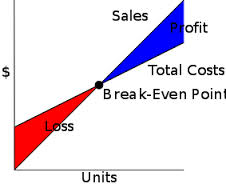Accounting

# What is Meant by Break Even Point?What is Meant by Break Even Point?

Break-even analysis is a method used in business to calculate the the total or number of items that needs to be sold during a specific period  so that you are able to cover what it costs to operate your business. Break-even analysis is dependent on three variable, the average per unit cost, the monthly fixed cost and the average per unit sales price.

The break even point is the level at which total sales are equal to total costs. Break-even analysis is a critical tool that allows managers to understand the relationship between prices, volume, and costs. This knowledge affects decisions about cost structure, pricing strategy, and which products go to market. Finding the break-even point begins with determining a product’s contribution margin, calculated by subtracting variable costs from revenues. Contribution margin can be expressed in dollars (to find break-even in units) or as a percentage ratio (for break-even in dollars). Total fixed costs are then divided by the contribution margin to find the break-even point.